# Two best ways to convert numbers to words in Excel

In this article I will show you two quick and free ways to convert currency numbers into English words in Excel 2019, 2016, 2013 and other versions.

Microsoft Excel is a great program to calculate this and that. It was initially developed to process large data arrays. However, it also lets creating accounting records like invoices, evaluation or balance sheets quickly and effectively.

In more or less solid payment documents it is necessary to duplicate numeric values with their word form. It is much harder to falsify typed numbers than those written by hand. Some swindler can try to make 8000 out of 3000, while it is almost impossible to secretly replace "three" with "eight".

So what you need is not just convert numbers to words in Excel (e.g. 123.45 to "one hundred and twenty three, forty five"), but spell out dollars and cents (e.g. \$29.95 as "twenty nine dollars and ninety nine cents" ), pounds and pence for GBP, euros and eurocents for EUR, etc.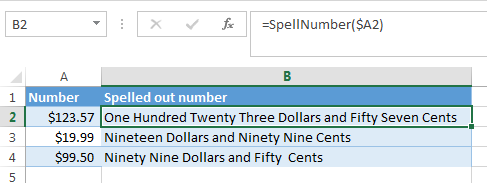Even the latest versions of Excel don't have a built-in tool for spelling numbers, not to mention earlier versions. But that is when Excel is really good. You can always improve its functionality using formulas in all their
combinations, VBA macros, or third-party add-ins.

Below you'll find two ways to convert numbers from figures to words

And, possibly, you may need to convert Words to Numbers in Excel

Note. If you are looking for the number to text conversion, which means you want Excel to see your number as text, it's a bit different thing. For this, you can use the TEXT function or a few other ways described in How to change numbers to text in Excel.

## SpellNumber VBA macro to convert numbers to words

As I have already mentioned, Microsoft didn't want to add a tool for this task. However, when they saw how many users needed it, they created and published the special VBA macro on their website. The macro does what its name SpellNumber suggests. All other macros I came across are based on the Microsoft code.

You can find the macro mentioned as "spellnumber formula". However, it is not a formula, but a macro function, or to be more precise Excel User defined function (UDF).

The spellnumber option is able to write dollars and cents. If you need a different currency, you can change "dollar" and "cent" with the name of your one.

If you are not a VBA savvy guy, below you will find a copy of the code. If you still don't want or haven't time to sort this out, please use this solution.

1. Open the workbook where you need to spell the numbers.
2. Press Alt+F11 to open the Visual Basic editor window.
3. If you have several books opened, check that the needed workbook is active using the list of projects in the upper left corner of the editor (one of the workbook elements is highlighted with blue).
4. In the editor menu go to Insert-> Module.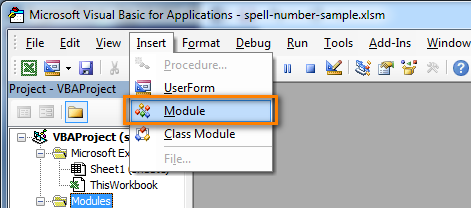5. You should see a window named YourBook - Module1. Select all of the code in the frame below and paste it to this window.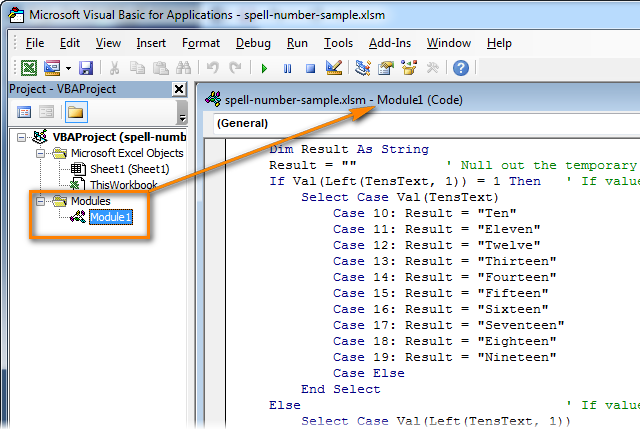6. Press Ctrl+S to save the updated workbook.

You will need to resave your workbook. When you try to save the workbook with a macro you'll get the message "The following features cannot be saved in macro-free workbook"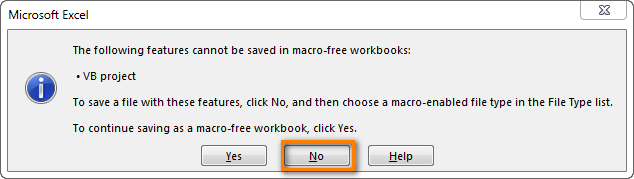Click No. When you see a new dialog, chose the Save as option. In the field "Save as type" pick the option "Excel macro-enabled workbook".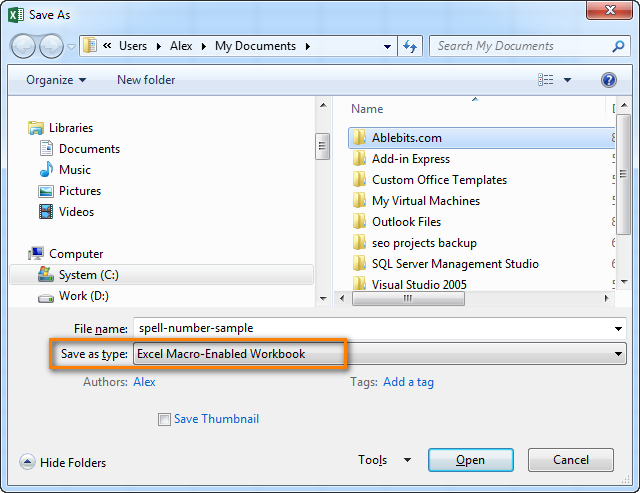#### Use SpellNumber macro in your worksheets

Now you can use the function SpellNumber in your Excel documents. Enter `=SpellNumber(A2)` into the cell where you need to get the number written in words. Here A2 is the address of the cell with the number or amount.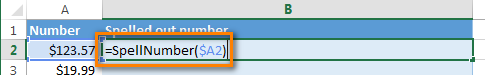Here you can see the result: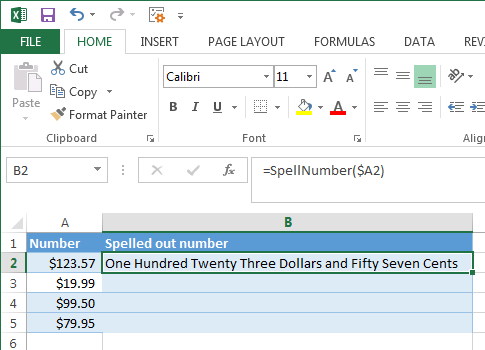Voila!

#### Quickly copy the SpellNumber function to other cells.

If you need to convert the entire table, not just 1 cell, place your mouse cursor to the lower right corner of the cell with the formula until it turns into a small black cross: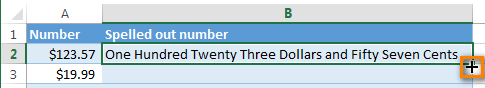Left-click and drag it across the column to fill in the formula. Release the button to see the results: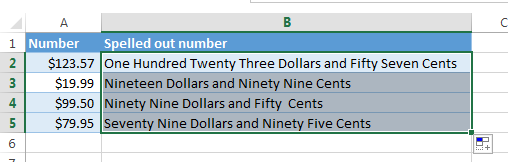Note. Please keep in mind that if you use SpellNumber with a link to another cell, the written sum will be updated each time the number in the source cell is changed.
You can also enter the number directly into the function, for example, `=SpellNumber(29.95)` (29.95 - without quotation marks and the Dollar sign).

#### Disadvantages of using macro to spell numbers in Excel

First off, you must know VBA to modify the code according to your needs. It is necessary to paste the code for each workbook, where you plan to change it. Otherwise, you will need to create a template file with macros and configure Excel to load this file at each start.

The main disadvantage of using a macro is if you send the workbook to somebody else, this person will not see the text unless the macro is built into the workbook. And even if it's built-in, they will get an alert that there are macros in the workbook.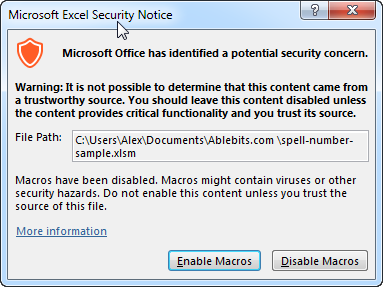## Spell numbers into words using a special add-in

For Excel users who need to quickly spell sums but don't have time to learn VBA or figure out workarounds, we created a special tool that can quickly perform the amount-to-words conversion for a few popular currencies. Please meet the Spell Number add-in included with the latest release of our Ultimate Suite for Excel.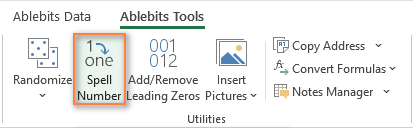Besides being ready for use, the tool is really flexible in converting amounts to text:

• You can select one of the following currencies: USD, EUR, GBP, BIT, AUD.
• Spell the fractional part in cents, pennies, or bitcents.
• Choose any text case for the result: lower case, UPPER CASE, Title Case, or Sentence case.
• Spell the decimal part in different ways.
• Include or omit zero cents.

The add-in supports all modern versions including Excel 365, Excel 2029, Excel 2016, Excel 2013, and Excel 2010. Please feel free to explore other capabilities on the product's home page linked above.

And now, let's see this number spelling utility in action:

1. Select an empty cell for the result.
2. On the Ablebits tab, in the Utilities group, click Spell Number.
3. In the Spill Number dialog window that appears, configure the following things:
• For the Select your number box, pick the cell containing the amount you want to get written as text.
• Specify the desired currently, letter case and the way the decimal part of the number should be spelled.
• Define whether to include zero cents or not.
• Choose whether to insert the result as a value or formula.
4. At the bottom of the dialog window, preview the result. If you are happy with the way your number is written, click Spell. Otherwise, try different settings.

The screenshot below demonstrates the default choices and the spelled number in B2. Please notice a formula (more precisely, a user-defined function) in the formula bar: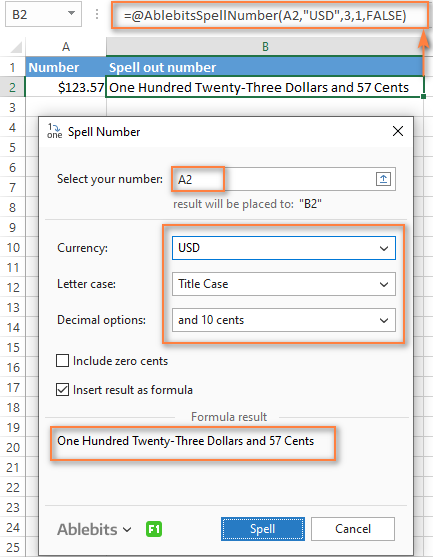And this is a quick demonstration of how other currencies can be spelled out: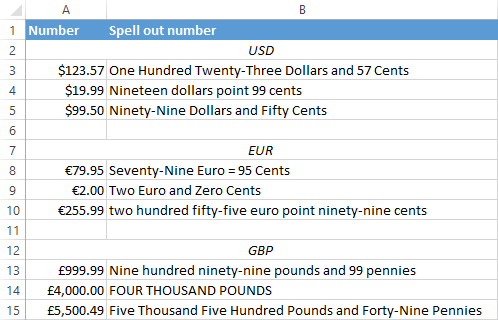Tips and notes:

• Because the Spell Number add-in was designed to handle real-life use cases such as invoices and other financial documents, it can only convert one number at a time.
• To spell a column of numbers, insert a formula in the first cell, and then copy the formula down.
• If there is chance that your source data may change in the future, it's best to insert the result as formula, so it updates automatically as the original number changes.
• When choosing the result as formula option, a custom user-defined function (UDF) is inserted. If you plan to share your workbook with someone who does not have the Ultimate Suite installed, remember to replace formulas with values before sharing.

## Reverse conversion - English words into numbers

Frankly, I can't imagine why you may need it. Just in case… :)

It appears that Excel MVP, Jerry Latham, created such Excel User defined function (UDF) as WordsToDigits. It converts English words back to number.

You can download Jerry's WordsToDigits workbook to see the UDF code. Here you'll also find his examples of how to use the function.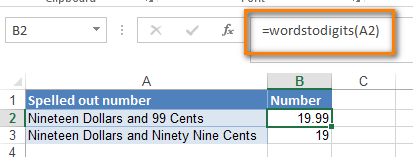You can see how the function works on the sheet "Sample Entries", where you will also be able to enter your own examples. If you plan to employ WordsToDigits in your documents, please be informed that this function has restrictions. For example, it doesn't recognize fractions entered in words. You will find all the details on the "Information" sheet.

## You may also be interested in

1. Thanks for the Awesome Tool.
I was unable to Convert a Number say for example QATARI RIYAL 1700/- In to QATARI RIYAL ONE THOUSAND SEVEN HUNDRED ONLY. I didn't see any option to do like this. Please, Help.

• Hi! Your example is a text. Extract a number from the text string using these guidelines. Replace the currency name in the VBA code and convert the number to text.

2. Nice

3. When I enter 1,200 it only displays "One thousand"

4. I used the VBA macro. Initially, it works fine. But after closing the Excel file, when I re-open it, the function doesn't work. It gives "#NAME" error. Any help?

5. Hi
=AEDtext(E23)

The above Function is working in my excel right now but when I open a new page in the same work book this function is not working. Kindly help me out

6. I get the result as : Sometimes it appears as Ambiguity and sometimes as syntax error.Please help.

7. Thanks for the Awesome Tool.
But,
I was unable to Convert a Number say for example 125.50/- in INR (Indian Rupee) to One Hundred and Twenty Five Rupees and Fifty Paisa. I didn't see any option to do like this. Please, help.

• Hi! You can replace the text "Dollars" with "Rupees" in the VBA code yourself.

8. Hi,

would like to ask the macro works at the first time, but when I closed and re-open the file, it doesn't work. it shows #NAME?

How to resolve this issue?

Regards,

9. I activated the spell function using the given module, now i want the currency to be in OMR not dollar, please assist.

10. I used the copy and paste code for SpellNumber; followed the instructions but when the "=SpellNumber(a4)" is entered, I get an error saying, Microsoft Visual Basic for Application, Compile error, syntax error. Is there something that I am not doing correctly or additional steps needed to be perform in Excel for the SpellNumber to work?

• Hello! There were a few unnecessary characters in the code. Try to copy and paste code into your file again.

• Thank you so much. It worked!! I am so excited! I appreciate what you are doing. Take care and God bless.

11. Hi sir, the spell number working is only current excel sheet and why it was not working by every work sheet and also old using excel sheets.

• Hi! The custom user-defined function works on the sheet in whose code it is inserted. Try to follow the recommendations from this article: Calling UDF from other workbooks. Hope you’ll find this information helpful.
Try to copy and paste the function code again.

• It doesn't work in any sheet. I followed the instructions as presented, but still got the syntax error/Compile error. When the function is entered in excel, e.g."=spellNumber(b3)". the error message appears and the following macro line is highlighted: Cents = GetTens(Left(Mid(MyNumber, DecimalPlace + 1) & _
"00", 2))

• Hi! Try again to copy and paste the function code into your workbook. There may have been an accidental copying error. I think this will help.

12. how to set excel formulas number to word in Lebanese pound

13. Good day po! I am using Bahrain Dinar currency for coverting number to word. And I have a little problem when it have a cents or "fils" in Bahrain. I manage to convert ten fils but what I need is hundred fils. I rarely see a tutorial on internet that has a Bahrain Dinar currency topic. US Dollars is the common so I don't have any idea on how to make a format for hundred fils which is in Bahrain Dinar. Until I found your link in google and I am really hoping that you can help me with my concern. Thank you in advance. I am looking forward to hearing from you. Have a nice weekend.

14. How to set excel formulas number to word in Philippine Peso?

15. Hi Sir,

I would like to have a formula to convert the Bahraini Dinar (BD) as 37.285 (THIRTY SEVEN AND FILS 285/1000 ONLY)

Thanks

Eg: Two thousand and cents ninety two

Thanks,

17. Hi experts,
I'm looking for a BVA code to convert numbers into words without showing currency and 3 decimal values. i.e. 10,550,125,589.325 (Ten Billion Five Hundred Fifty Million One Hundred Twenty-Five Thousand Five Hundred Eighty-Nine And 325/1000 only).
Thank you
Dipak

18. For rupees not working

19. Sir, I have converted 1056 and the result is one thousand fifty-six only. I was wondering if there's any way to make it like this:

One Thousand and Fifty-Six Only

20. hello sir thank you so much for this but I do have an inquiry instead of converting the cents to words how to make it stay as figure only?

One Hundred Ninety Pesos and 87 /100 Only

Select Case Cents
Case ""
Cents = " Only"
Case "One"
Cents = " and One Cent"
Case Else
Cents = " and " & Cents & " /100 Only"
End Select

21. How to set as below format:

For example:

RM 3,600.00
in Words: ***THREE THOUSAND SIX HUNDRED ONLY***

22. Brilliant.

??

23. Thanks for sharing this.
This is very helpful in excel for me.
But still i have one query whenever i am opening a new excel, i need to insert module formula's in each excel and need to save every time to each excel. Is there any solution for my solution, because i don't want to save this module formula for each excel many times.

• Hello!
Try saving your custom function in an add-in file as described in this tutorial.

24. How can make it up to 3 decimals, currently it is only 2 desimals, I want it instead of 0.39 thirty nine to be three hundred ninty one

• Hi!
To get 0.391 in cell, change the number format as described in this guide.
You may also need to apply to round the number to 3 decimal places.
Hope this is what you need.

25. Amazing!
Thank you so much!

26. Actually all the formula / function don't work in excel program in Android mobile.
As the formula given requires Add In / VBA.
I require a simple formula to convert Number in Words in Indian method
e.g. Rs 99,99 999.99 should be in Words "Rupees Ninety Nine Lakh Ninety Nine Thousand Nine Hundred Ninety Nine Paise Ninety Nine Only"
EXPECTING A FAVORABLE HELP.

27. Thank you for this! Big Help!

28. dears,

please need this macro without currency :)

thanks

29. Hi mine is working perfectly! thank you
but having issue sometime (Seventeen Dollars and Thirty Two Cents) but excel numeric digit show 17.33 anyone help me to find the solution or having only option to change the decimal places 2 to 3

• Hello!
Your number is the result of a calculation. If you increase the number of visible decimal places in the cell, you will see 17.3268 or something similar. Excel automatically rounds the number it shows you on the worksheet. But the value in the cell does not change. The SpellNumber function does not round a number. To make the data match, use the ROUND function in your formula.

• Sir Alexander Trifuntov

Please help me on this I am using the below VBA code as a result I get the word "Rupees" at the beginning of the result and the word "Only" at the end.

Example
123
=Spell Number(One Hundred and Twenty Three )
Need Result
Rupees One Hundred and Twenty Three Only

(Using Below Code)

Option Explicit
Function SpellNumber(ByVal MyNumber)
Dim uStr As String
Dim uFNum As Integer
Dim uStrPoint
Dim uStrNumber
Dim uPoint As String
Dim uNumber As String
Dim uP() As Variant
Dim uDP
Dim uCnt As Integer
Dim uResult, uT As String
Dim uLen As Integer
On Error Resume Next
uP = Array("", "Thousand ", "Million ", "Billion ", "Trillion ", " ", " ", " ", " ")
uNumber = Trim(Str(MyNumber))
uDP = InStr(uNumber, ".")
uPoint = ""
uStrNumber = ""
If uDP > 0 Then
uPoint = " point "
uStr = Mid(uNumber, uDP + 1)
uStrPoint = Left(uStr, Len(uNumber) - uDP)
For uFNum = 1 To Len(uStrPoint)
uStr = Mid(uStrPoint, uFNum, 1)
uPoint = uPoint & Digits(uStr) & " "
Next uFNum
uNumber = Trim(Left(uNumber, uDP - 1))
End If
uCnt = 0
uResult = ""
uT = ""
uLen = 0
uLen = Int(Len(Str(uNumber)) / 3)
If (Len(Str(uNumber)) Mod 3) = 0 Then uLen = uLen - 1
Do While uNumber ""
If uLen = uCnt Then
uT = HundredsDigits(Right(uNumber, 3), False)
Else
If uCnt = 0 Then
uT = HundredsDigits(Right(uNumber, 3), True)
Else
uT = HundredsDigits(Right(uNumber, 3), False)
End If
End If
If uT "" Then
uResult = uT & uP(uCnt) & uResult
End If
If Len(uNumber) > 3 Then
uNumber = Left(uNumber, Len(uNumber) - 3)
Else
uNumber = ""
End If
uCnt = uCnt + 1
Loop
uResult = uResult & uPoint
SpellNumber = uResult
End Function
Function HundredsDigits(uHDgt, uB As Boolean)
Dim uRStr As String
Dim uStrNum As String
Dim uStr As String
Dim uI As Integer
Dim uBB As Boolean
uStrNum = uHDgt
uRStr = ""
On Error Resume Next
uBB = True
If Val(uStrNum) = 0 Then Exit Function
uStrNum = Right("000" & uStrNum, 3)
uStr = Mid(uStrNum, 1, 1)
If uStr "0" Then
uRStr = Digits(Mid(uStrNum, 1, 1)) & "Hundred "
Else
If uB Then
uRStr = "and "
uBB = False
Else
uRStr = " "
uBB = False
End If
End If
If Mid(uStrNum, 2, 2) "00" Then
uRStr = uRStr & TenDigits(Mid(uStrNum, 2, 2), uBB)
End If
HundredsDigits = uRStr
End Function
Function TenDigits(uTDgt, uB As Boolean)
Dim uStr As String
Dim uI As Integer
Dim uArr_1() As Variant
Dim uArr_2() As Variant
Dim uT As Boolean
uArr_1 = Array("Ten ", "Eleven ", "Twelve ", "Thirteen ", "Fourteen ", "Fifteen ", "Sixteen ", "Seventeen ", "Eighteen ", "Nineteen ")
uArr_2 = Array("", "", "Twenty ", "Thirty ", "Forty ", "Fifty ", "Sixty ", "Seventy ", "Eighty ", "Ninety ")
uStr = ""
uT = True
On Error Resume Next
If Val(Left(uTDgt, 1)) = 1 Then
uI = Val(Right(uTDgt, 1))
If uB Then uStr = "and "
uStr = uStr & uArr_1(uI)
Else
uI = Val(Left(uTDgt, 1))
If Val(Left(uTDgt, 1)) > 1 Then
If uB Then uStr = "and "
uStr = uStr & uArr_2(Val(Left(uTDgt, 1)))
uT = False
End If
If uStr = "" Then
If uB Then
uStr = "and "
End If
End If
If Right(uTDgt, 1) "0" Then
uStr = uStr & Digits(Right(uTDgt, 1))
End If
End If
TenDigits = uStr
End Function
Function Digits(uDgt)
Dim uStr As String
Dim uArr_1() As Variant
uArr_1 = Array("Zero ", "One ", "Two ", "Three ", "Four ", "Five ", "Six ", "Seven ", "Eight ", "Nine ")
uStr = ""
On Error Resume Next
uStr = uArr_1(Val(uDgt))
Digits = uStr
End Function

30. hello all genius,
can anyone help me?

100000 ... when i tried this code it is coming "one hundred thousand".

i need it as "one lakh"/ or "Point million"

how can i add this extra requirement??

31. SO Helping ... Specially by pics....

32. I don't know what to say. This worked for me like magic. I was looking for this for hours and at last, I found you. WOW! Let me salute you, Mr. Admin. Thank you very much.

34. Hi there,

As far as VBA is often disabled for security reasons, I have written the FORMULA which spells sums up to 999 billion dollars, e.g.:

907 654 000 012,99
nine hundred and seven billion six hundred and fifty four million twelve dollars and 99 cents

The formula is sensitive to Zero thousands/mln/bln (omits thousands/mln/bln if they are 000)
Also spells "dollar" or "dollars" depending if the last digit of dollars is 1 or more (21 dollar, and 17 dollars)

Simply replace "A1" in tjhe code with the name of the cell you need (better with Ctrl-H)

---

=CONCATENATE(IF(INT(A1/1000000000)>=1;CONCATENATE(TRIM(CONCATENATE(IF(INT(A1/1000000000)>99;CONCATENATE(CHOOSE(INT(INT(A1/1000000000)/100);"one";"two";"three";"four";"five";"six";"seven";"eight";"nine");" hundred ");"");IF(AND(INT(A1/1000000000)>100;MOD(INT(A1/1000000000);100)>0);"and ";"");IF(MOD(INT(A1/1000000000);100)=0;"";IF(MOD(INT(A1/1000000000);100)=1;CONCATENATE(TRIM(CONCATENATE(IF(INT(MOD(A1;1000000000)/1000000)>99;CONCATENATE(CHOOSE(INT(INT(MOD(A1;1000000000)/1000000)/100);"one";"two";"three";"four";"five";"six";"seven";"eight";"nine");" hundred ");"");IF(AND(INT(MOD(A1;1000000000)/1000000)>100;MOD(INT(MOD(A1;1000000000)/1000000);100)>0);"and ";"");IF(MOD(INT(MOD(A1;1000000000)/1000000);100)=0;"";IF(MOD(INT(MOD(A1;1000000000)/1000000);100)=1;CONCATENATE(TRIM(CONCATENATE(IF(INT(MOD(A1;1000000)/1000)>99;CONCATENATE(CHOOSE(INT(INT(MOD(A1;1000000)/1000)/100);"one";"two";"three";"four";"five";"six";"seven";"eight";"nine");" hundred ");"");IF(AND(INT(MOD(A1;1000000)/1000)>100;MOD(INT(MOD(A1;1000000)/1000);100)>0);"and ";"");IF(MOD(INT(MOD(A1;1000000)/1000);100)=0;"";IF(MOD(INT(MOD(A1;1000000)/1000);100)=1;CONCATENATE(TRIM(CONCATENATE(IF(INT(MOD(A1;1000))>99;CONCATENATE(CHOOSE(INT(INT(MOD(A1;1000))/100);"one";"two";"three";"four";"five";"six";"seven";"eight";"nine");" hundred ");"");IF(AND(INT(MOD(A1;1000))>100;MOD(INT(MOD(A1;1000));100)>0);"and ";"");IF(MOD(INT(MOD(A1;1000));100)=0;"";IF(MOD(INT(MOD(A1;1000));100)<20;CHOOSE(MOD(INT(MOD(A1;1000));100);"one";"two";"three";"four";"five";"six";"seven";"eight";"nine";"ten";"eleven";"twelve";"thirteen";"fourteen";"fifteen";"sixteen";"seventeen";"eighteen";"nineteen");CONCATENATE(CHOOSE(INT(MOD(INT(MOD(A1;1000));100)/10);"ERROR";"twenty";"thirty";"forty";"fifty";"sixty";"seventy";"eighty";"ninety";"MORE");CHOOSE(INT(MOD(INT(MOD(A1;1000));10))+1;"";" one";" two";" three";" four";" five";" six";" seven";" eight";" nine"))))));" ");"");IF(INT(MOD(A1;10))=1;"dollar and ";"dollars and ");ROUND(MOD(A1;1)*100;0);" cents")

• Sorry, the code somehow ripped.
Write once more:

=CONCATENATE(IF(INT(A1/1000000000)>=1;CONCATENATE(TRIM(CONCATENATE(IF(INT(A1/1000000000)>99;CONCATENATE(CHOOSE(INT(INT(A1/1000000000)/100);"one";"two";"three";"four";"five";"six";"seven";"eight";"nine");" hundred ");"");IF(AND(INT(A1/1000000000)>100;MOD(INT(A1/1000000000);100)>0);"and ";"");IF(MOD(INT(A1/1000000000);100)=0;"";IF(MOD(INT(A1/1000000000);100)=1;CONCATENATE(TRIM(CONCATENATE(IF(INT(MOD(A1;1000000000)/1000000)>99;CONCATENATE(CHOOSE(INT(INT(MOD(A1;1000000000)/1000000)/100);"one";"two";"three";"four";"five";"six";"seven";"eight";"nine");" hundred ");"");IF(AND(INT(MOD(A1;1000000000)/1000000)>100;MOD(INT(MOD(A1;1000000000)/1000000);100)>0);"and ";"");IF(MOD(INT(MOD(A1;1000000000)/1000000);100)=0;"";IF(MOD(INT(MOD(A1;1000000000)/1000000);100)=1;CONCATENATE(TRIM(CONCATENATE(IF(INT(MOD(A1;1000000)/1000)>99;CONCATENATE(CHOOSE(INT(INT(MOD(A1;1000000)/1000)/100);"one";"two";"three";"four";"five";"six";"seven";"eight";"nine");" hundred ");"");IF(AND(INT(MOD(A1;1000000)/1000)>100;MOD(INT(MOD(A1;1000000)/1000);100)>0);"and ";"");IF(MOD(INT(MOD(A1;1000000)/1000);100)=0;"";IF(MOD(INT(MOD(A1;1000000)/1000);100)=1;CONCATENATE(TRIM(CONCATENATE(IF(INT(MOD(A1;1000))>99;CONCATENATE(CHOOSE(INT(INT(MOD(A1;1000))/100);"one";"two";"three";"four";"five";"six";"seven";"eight";"nine");" hundred ");"");IF(AND(INT(MOD(A1;1000))>100;MOD(INT(MOD(A1;1000));100)>0);"and ";"");IF(MOD(INT(MOD(A1;1000));100)=0;"";IF(MOD(INT(MOD(A1;1000));100)<20;CHOOSE(MOD(INT(MOD(A1;1000));100);"one";"two";"three";"four";"five";"six";"seven";"eight";"nine";"ten";"eleven";"twelve";"thirteen";"fourteen";"fifteen";"sixteen";"seventeen";"eighteen";"nineteen");CONCATENATE(CHOOSE(INT(MOD(INT(MOD(A1;1000));100)/10);"ERROR";"twenty";"thirty";"forty";"fifty";"sixty";"seventy";"eighty";"ninety";"MORE");CHOOSE(INT(MOD(INT(MOD(A1;1000));10))+1;"";" one";" two";" three";" four";" five";" six";" seven";" eight";" nine"))))));" ");"");IF(INT(MOD(A1;10))=1;"dollar and ";"dollars and ");ROUND(MOD(A1;1)*100;0);" cents")

• sorry again, the code rips while publishing... half the text simply disappears :(

35. Hi Friends,

Could you tell me the code where I can mention "and" before the last figures e.g. Rs 1,987,290
(Rupees one million nine Hundred eighty seven thousand two hundred and ninety only)

36. Hi,

First of all thanks for the wonderful formulas. May I get the formula for UAE dirham and Indian Rupees as well